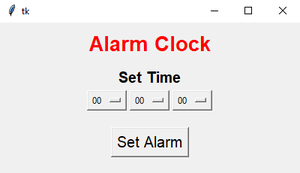# Create an Alarm Clock using Tkinter

• Difficulty Level : Easy
• Last Updated : 19 Oct, 2021

Prerequisite: Python GUI – tkinter, winsound, time, and datetime.

As we all know, nowadays wake up on time is a very difficult task. The solution is the Alarm Clock. In this article, we will learn, How to create an Alarm Clock using Tkinter in Python. Without alarms, several people would oversleep and land up late for work. Alarm clocks may also be useful for keeping sleep schedules regular.

• Tkinter: Python offers multiple choices for developing a GUI (Graphical User Interface). Out of all the GUI strategies, tkinter is that the most ordinarily used technique. It’s a customary Python interface to the Tk GUI toolkit shipped with Python.
• Winsound: The winsound module provides access to the essential sound-playing machinery provided by Windows platforms. It includes functions and a number of other constants. Beep the PC’s speaker.
• time: Time module in Python provides varied time-related functions. This module comes with Python’s normal modules.
• datetime: The main focus of datetime is to form it simpler to access attributes of the thing associated with dates, times, and time zones.

Below is what GUI looks like:-Let’s Understand step by step implementation:

Step1: Import Required Library

## Python3

 `# Import Required Library``from` `tkinter ``import` `*``import` `datetime``import` `time``import` `winsound`

Syntax:

# Button

Button(Object Name, text=”Enter Text”,**attr)

# Label

Label(Object Name, text=”Enter Text”, command=”Enter Command” , **attr)

# Frame

Frame(Object Name, **attr)

OptionMenu(“Object Name”, “Data Type”, “list of value in form of tuple”, **attr)

We will create a three-option menu:-

• Hours (00–24)
• Minutes (00–60)
• Seconds (00–60)

Time is in 24-hour time format.

Step 3: Make a function named alarm(), which performs alarm clock work.

## Python3

 `def` `alarm():``    ``# Infinite Loop``    ``while` `True``:``        ``# Set Alarm``        ``set_alarm ``=` `f``"{hour.get()}:{minute.get()}:{second.get()}"` `        ``# Wait for one seconds``        ``time.sleep(``1``)` `        ``# Get current time``        ``current_time ``=` `datetime.datetime.now().strftime(``"%H:%M:%S"``)` `        ``# Check whether set alarm is equal to current time or not``        ``if` `current_time ``=``=` `set_alarm:``            ``print``(``"Time to Wake up"``)``            ``# Playing sound``            ``winsound.PlaySound(``"sound.wav"``,winsound.SND_ASYNC)`

Below is the full implementation:

• Make Infinite Loop
• Get hours, minutes, seconds value from the user
• Wait for one second using time module
• Get Current time using datetime module
• Check if the current time is equal to set time; play sound using winsound module

## Python3

 `# Import Required Library``from` `tkinter ``import` `*``import` `datetime``import` `time``import` `winsound``from` `threading ``import` `*` `# Create Object``root ``=` `Tk()` `# Set geometry``root.geometry(``"400x200"``)` `# Use Threading``def` `Threading():``    ``t1``=``Thread(target``=``alarm)``    ``t1.start()` `def` `alarm():``    ``# Infinite Loop``    ``while` `True``:``        ``# Set Alarm``        ``set_alarm_time ``=` `f``"{hour.get()}:{minute.get()}:{second.get()}"` `        ``# Wait for one seconds``        ``time.sleep(``1``)` `        ``# Get current time``        ``current_time ``=` `datetime.datetime.now().strftime(``"%H:%M:%S"``)``        ``print``(current_time,set_alarm_time)` `        ``# Check whether set alarm is equal to current time or not``        ``if` `current_time ``=``=` `set_alarm_time:``            ``print``(``"Time to Wake up"``)``            ``# Playing sound``            ``winsound.PlaySound(``"sound.wav"``,winsound.SND_ASYNC)` `# Add Labels, Frame, Button, Optionmenus``Label(root,text``=``"Alarm Clock"``,font``=``(``"Helvetica 20 bold"``),fg``=``"red"``).pack(pady``=``10``)``Label(root,text``=``"Set Time"``,font``=``(``"Helvetica 15 bold"``)).pack()` `frame ``=` `Frame(root)``frame.pack()` `hour ``=` `StringVar(root)``hours ``=` `(``'00'``, ``'01'``, ``'02'``, ``'03'``, ``'04'``, ``'05'``, ``'06'``, ``'07'``,``         ``'08'``, ``'09'``, ``'10'``, ``'11'``, ``'12'``, ``'13'``, ``'14'``, ``'15'``,``         ``'16'``, ``'17'``, ``'18'``, ``'19'``, ``'20'``, ``'21'``, ``'22'``, ``'23'``, ``'24'``        ``)``hour.``set``(hours[``0``])` `hrs ``=` `OptionMenu(frame, hour, ``*``hours)``hrs.pack(side``=``LEFT)` `minute ``=` `StringVar(root)``minutes ``=` `(``'00'``, ``'01'``, ``'02'``, ``'03'``, ``'04'``, ``'05'``, ``'06'``, ``'07'``,``           ``'08'``, ``'09'``, ``'10'``, ``'11'``, ``'12'``, ``'13'``, ``'14'``, ``'15'``,``           ``'16'``, ``'17'``, ``'18'``, ``'19'``, ``'20'``, ``'21'``, ``'22'``, ``'23'``,``           ``'24'``, ``'25'``, ``'26'``, ``'27'``, ``'28'``, ``'29'``, ``'30'``, ``'31'``,``           ``'32'``, ``'33'``, ``'34'``, ``'35'``, ``'36'``, ``'37'``, ``'38'``, ``'39'``,``           ``'40'``, ``'41'``, ``'42'``, ``'43'``, ``'44'``, ``'45'``, ``'46'``, ``'47'``,``           ``'48'``, ``'49'``, ``'50'``, ``'51'``, ``'52'``, ``'53'``, ``'54'``, ``'55'``,``           ``'56'``, ``'57'``, ``'58'``, ``'59'``, ``'60'``)``minute.``set``(minutes[``0``])` `mins ``=` `OptionMenu(frame, minute, ``*``minutes)``mins.pack(side``=``LEFT)` `second ``=` `StringVar(root)``seconds ``=` `(``'00'``, ``'01'``, ``'02'``, ``'03'``, ``'04'``, ``'05'``, ``'06'``, ``'07'``,``           ``'08'``, ``'09'``, ``'10'``, ``'11'``, ``'12'``, ``'13'``, ``'14'``, ``'15'``,``           ``'16'``, ``'17'``, ``'18'``, ``'19'``, ``'20'``, ``'21'``, ``'22'``, ``'23'``,``           ``'24'``, ``'25'``, ``'26'``, ``'27'``, ``'28'``, ``'29'``, ``'30'``, ``'31'``,``           ``'32'``, ``'33'``, ``'34'``, ``'35'``, ``'36'``, ``'37'``, ``'38'``, ``'39'``,``           ``'40'``, ``'41'``, ``'42'``, ``'43'``, ``'44'``, ``'45'``, ``'46'``, ``'47'``,``           ``'48'``, ``'49'``, ``'50'``, ``'51'``, ``'52'``, ``'53'``, ``'54'``, ``'55'``,``           ``'56'``, ``'57'``, ``'58'``, ``'59'``, ``'60'``)``second.``set``(seconds[``0``])` `secs ``=` `OptionMenu(frame, second, ``*``seconds)``secs.pack(side``=``LEFT)` `Button(root,text``=``"Set Alarm"``,font``=``(``"Helvetica 15"``),command``=``Threading).pack(pady``=``20``)` `# Execute Tkinter``root.mainloop()`

Output:

My Personal Notes arrow_drop_up